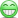# VRM Credit Risk Chapter 6

#### alexwallace

##### Member
Hi David @David Harper CFA FRM

I came across the following exercise:which has the solutionThis is a simple question but one thing drove me mad (I do not exclude the opportunity my mind went blank due to being tired) But anyway, I calculated variance in 6.11 as E(L^2) - {E(L)}^2 and
E(L^2) = 0.995*0^2 + 0.005* { 1,000,000 * 0.6}^2
Then Variance = 1,791,000 ----------> st.dev = sqrt(1,791,000) = 42,320 ----> this part coincides with the answer an this is OK.

However, when I try to calculate portfolio variance with 3 similar loans I get:Variance = 3*1,791,000 * {1 + 2*0.2} = 7,522,200 (and this also coincides with book , where it is 0.007522

#### David Harper CFA FRM

##### David Harper CFA FRM
Staff member
Subscriber
Hi @alexwallace Today I took a fresh look at 6.13, and I finally get what they are doing but it's just an awkward (if not inaccurate) way to phrase the question. This concept is really based on prior years' Nocco & Stulz reading, so question 6.13 should be more like:
• 6.13.a. If the company wants to target a rating of BBB, how much economic (or equity) capital should it hold (or carry)? Answer: Because a BBB-rated company has a one-year (conditional) PD of 0.18%, it should not breach (i.e., fall below) the default threshold with probability of 1 - 0.18% = 99.82% or confidence level. EC confidence level is 99.82% because the implied probability of "insolvency" is 0.18%.
• 6.13.a. If the company wants to target a rating of A, how much economic (or equity) capital should it hold (or carry)? Answer: Because a BBB-rated company has a one-year (conditional) PD of 0.06%, it should not breach (i.e., fall below) the default threshold with probability of 1 - 0.06% = 99.94% or confidence level. EC confidence level is 99.94% because the implied probability of "insolvency" is 0.06%.
Then you can see how they've taken this concept of "targeting the company to have an as-if rating" to phrase (a) and (b), but I think it's pretty weak. Thanks,